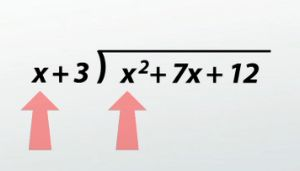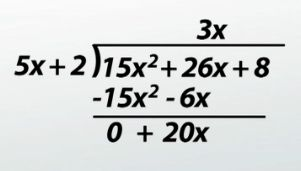# How to Divide Polynomials with Long Division

An error occurred trying to load this video.

Try refreshing the page, or contact customer support.

Coming up next: How to Use Synthetic Division to Divide Polynomials

### You're on a roll. Keep up the good work!

Replay
Your next lesson will play in 10 seconds
• 0:05 Polynomial Long Division
• 0:35 Example #1
• 2:56 Example #2
• 5:08 Example #3
• 7:25 Lesson Summary

Want to watch this again later?

Timeline
Autoplay
Autoplay
Speed

#### Recommended Lessons and Courses for You

Lesson Transcript
Instructor: Kathryn Maloney

Kathryn teaches college math. She holds a master's degree in Learning and Technology.

Arithmetic long division and polynomial long division are very similar. Yes, it's a long process, but once you have the rhythm you will get every problem correct!

## Polynomial Long Division

Polynomial long division is very similar to the long division we did as kids, except now we have numbers and xs. It takes only two steps that are repeated until you're done.

1. Divide the first terms.
2. Multiply the result from the divisor, then subtract it from the dividend.
3. Repeat!

Let's review the parts of a division problem. We have the divisor divided into the dividend, and of course our answer is the quotient.

## Example #1

Here's our first example: (x^2 + 7x + 12) / (x + 3).

To fill in our long division, x^2 + 7x + 12 is the dividend, so it goes under the long division symbol. x+3 is the divisor, so it goes to the outside, in front of the long division symbol. Now we're ready to start the division. The steps are the same every time. It's easier to show and talk about the steps than listing them, so let's take a look. To find the first term of the quotient, we take the first terms from the divisor and the dividend and divide them. I like to write them as a fraction; it's easier to divide or reduce: x^2 / x = x. x is the first term of the quotient; we write it above the long division symbol. To figure out what we will subtract from the dividend, we multiply x times the divisor, x+3: x(x+3) = x^2 + 3x. x^2 + 3x is written under the dividend, matching like terms. Here's where I like to make life easy. To subtract the polynomials, I simply change their signs and add! So x^2 -x^2 = 0, and 7x - 3x = 4x. Just like we did in long division, we bring down the next term, which is 12.Guess what? We do the exact same step! To find the next term of the quotient, we take the new first terms and divide them, so we'll have 4x / x. I like to write them as a fraction; it's easier: 4x / x = 4. 4, or positive 4, is the next term of the quotient. Remember, that gets written next to the x above the long division symbol. To figure out what we will subtract from the dividend, we multiply 4 times the divisor, (x + 3). (4)(x + 3) = 4x + 12. 4x + 12 is written under the dividend, matching like terms. Next, I like to change the signs and add down: 4x + 12 - 4x - 12 = 0. It turns out our remainder is zero. What does our answer look like? Just look above the division symbol: x + 4. Are you thinking to yourself 'That wasn't too bad'? Let's try one a little longer. Don't panic. Follow the exact same steps, repeat and we'll have an answer.

## Example #2

Divide (15x^2 + 26x + 8) / (5x + 2).

To fill in our long division, 15x^2 + 26x + 8 is in the dividend, so it goes under the long division symbol. 5x + 2 is the divisor, so it goes to the outside in the front of the long division symbol. Now we're ready to start the division. To find the first term of the quotient, we take the first terms from the divisor and dividend and divide them: 15x^2 / 5x = 3x. 3x is the first term of the quotient; we write it above the long division symbol. To figure out what we will subtract from the dividend, we multiply 3x times the divisor, 5x + 2: 3x(5x + 2) = 15x^2 + 6x. 15x^2 + 6x is written under the dividend, matching like terms. I like to change the signs and add straight down: 15x^2 - 15x^2 = 0, and 26x + (-6x) = 20x. Just like we did in long division, we're going to bring down the next term, which is 8.To unlock this lesson you must be a Study.com Member.

### Register to view this lesson

Are you a student or a teacher?

### Unlock Your Education

#### See for yourself why 30 million people use Study.com

##### Become a Study.com member and start learning now.
Back
What teachers are saying about Study.com

### Earning College Credit

Did you know… We have over 160 college courses that prepare you to earn credit by exam that is accepted by over 1,500 colleges and universities. You can test out of the first two years of college and save thousands off your degree. Anyone can earn credit-by-exam regardless of age or education level.